CATEGORIES:

# The eccentricity and directrix of an ellipse. Consider the focal radii of an ellipse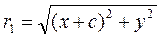;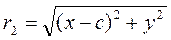.

By definition, we have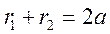.

Consider the difference of squares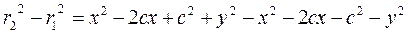;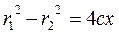,

or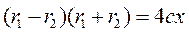.y

d1 М(х;у) d2r1 r2

F1(–c;0) 0 F2(c;0) x

x=–l x=l

to determine the focal radii, we solve the system of equations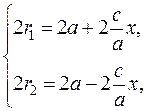orDefinition. The ratio of distances between the foci to the sum of focal radii is called eccentricity: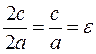.

If the distance between the foci is less than 2а, then the eccentricity is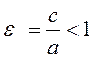.

Thus, the focal radii of the ellipse are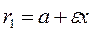,.

Definition. The directrix of an ellipse is the straight line parallel to the y-axis such that the ratio of the focal radius to the distance from an ellipse point to it is constant and equal the eccentricity.

Let us draw two straight lines x=–l and x=l parallel to the y-axis and find l such that the ratio of the focal radius to the distance from a point М to this straight line is constant and equals the eccentricity: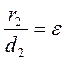.

Substituting the distance and the focal radius, we obtain.

The ratio is equal to the eccentricity when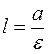, i.e.,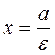is the directrix. By analogy, we obtain equations of the directrices: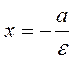;,

where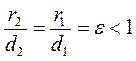.

Date: 2015-01-02; view: 1720

 <== previous page | next page ==> Ellipse | Tangents to an Ellipse
doclecture.net - lectures - 2014-2023 year. Copyright infringement or personal data (0.01 sec.)